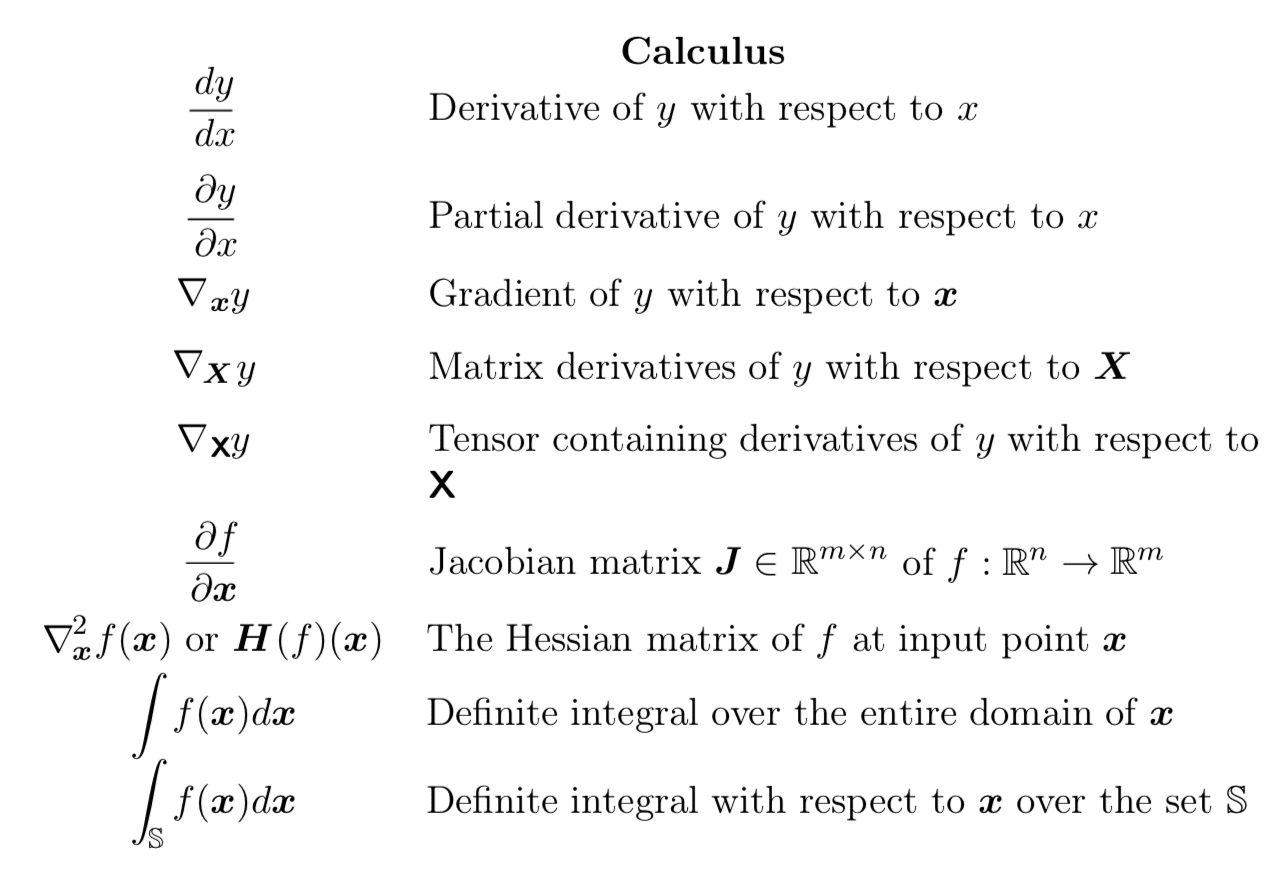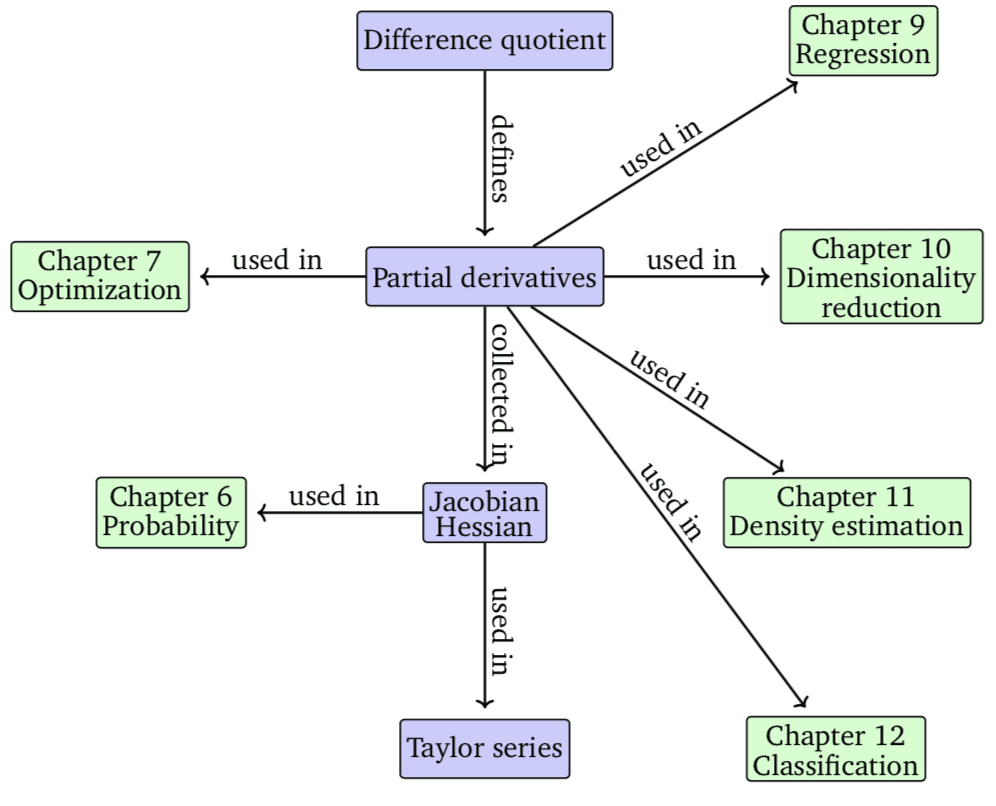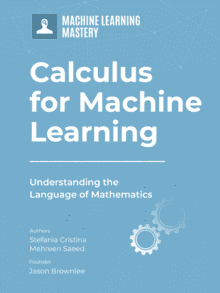# Calculus Books for Machine Learning

Last Updated on December 23, 2020

Knowledge of calculus is not required to get results and solve problems in machine learning or deep learning.

However, knowing some calculus will help you in a number of ways, such as in reading mathematical notation in books and papers, and in understanding the terms used to describe fitting models like “gradient,” and in understanding the learning dynamics of models fit via optimization such as neural networks.

Calculus is a challenging topic as taught at a university level, but you don’t need to know all of calculus, just a handful of terms and methods related to numerical function optimization, central to fitting algorithms like neural networks. And the best way to get a handle on calculus is from books.

In this tutorial, you will discover books on calculus for machine learning.

After completing this tutorial, you will know:

• Which books on machine learning provide a gentle introduction to the relevant calculus topics.
• Books that you can use to learn the intuitions, history, and techniques of calculus.
• Textbooks you can use for reference or deeper learning of calculus techniques and their proofs.

Let’s get started.Calculus Books for Machine Learning
Photo by Roanish, some rights reserved.

## Tutorial Overview

This tutorial is divided into three parts; they are:

1. Calculus in Machine Learning Books
2. Introductory Calculus Books
3. Calculus Textbooks

## Calculus in Machine Learning Books

This is perfect if you learned calculus in school (a long time ago) and need a refresh, or if you need a quick crash course in the terms and methods.

Many top machine learning and deep learning textbooks will cover the basics, and it is often enough for most cases, e.g. when you’re focused on getting results with machine learning algorithms.

Also, it helps if you already own a machine learning textbook that covers some calculus as you don’t need to get another book.

Two great textbooks that cover some calculus include:

The coverage of calculus in the “Deep Learning” textbook is brief.

Calculus is introduced in the context of optimization, first in terms of linear regression then more generally for multivariate optimization–seen when fitting neural nets.

This includes topics such as:

• Derivative
• Partial derivative
• Second derivative
• Hessian matrix
• Critical points
• Stationary points
• Local maximum
• Global minimum
• Jacobian matrix

And more.Calculus Terms.
Taken from Page xiii, Deep Learning, 2016.

The book “Pattern Recognition and Machine Learning” provides more in-depth coverage.

Specifically:

• Chapter 10: Approximate Inference
• Appendix D: Calculus of Variations

Appendix D introduces the topic of “calculus of variations” and Chapter 10 makes use of the technique. The topic is also covered in the deep learning book.

If a typical calculus problem involves finding a value of a variable that optimizes a function, then calculus of variations is about funding a function that optimizes another function. We can see that this is relevant in machine learning and specifically neural networks as the neural network model (circuit) learns arbitrary functions under a loss function.

A common problem in conventional calculus is to find a value of x that maximizes (or minimizes) a function y(x). Similarly, in the calculus of variations we seek a function y(x) that maximizes (or minimizes) a functional F [y]. That is, of all possible functions y(x), we wish to find the particular function for which the functional F [y] is a maximum (or minimum).

— Page 703, Pattern Recognition and Machine Learning, 2006.

The calculus of variations is not required to fit neural nets, but it provides a useful tool to better understand the problem we are solving when fitting a neural net and the types of learning dynamics we may see in practice.

Finally, we are starting to see books dedicated to the mathematical understanding that underlies machine learning.

One example is “Mathematics for Machine Learning.”

This book covers a lot of the calculus required for machine learning and provides the context showing where it fits in terms of the optimization (training/learning) of models.Calculus and its Connection to Machine Learning.
Taken from Mathematics for Machine Learning, page 140.

The discussion of calculus is confined to Chapter 5: Vector Calculus, which covers the following topics:

• Section 5.1 Differentiation of Univariate Functions
• Section 5.2 Partial Differentiation and Gradients
• Section 5.3 Gradients of Vector-Valued Functions
• Section 5.4 Gradients of Matrices
• Section 5.5 Useful Identities for Computing Gradients
• Section 5.6 Backpropagation and Automatic Differentiation
• Section 5.7 Higher-Order Derivatives
• Section 5.8 Linearization and Multivariate Taylor Series

This book is an excellent starting point to fill out or refresh your knowledge of calculus for machine learning.

## Introductory Calculus Books

Knowing the names of terms is one thing, but what if you want to know some of the methods more generally?

For that, I would recommend a solid beginner book, such as:

These are not textbooks; instead, they assume little or no background (e.g. pre-calculus) and will step you through the intuitions and the techniques and their application to simple exercises.

Intuition is key! We’re not doing math degrees; we’re solving machine learning problems.

A textbook will teach you the method and the proof but rarely tell you what problem the method was designed to solve in the first place and a little history. I think background is critical.

I own both books. I like “Calculus For Dummies” and would recommend it, if you can get past the name and style.

There are many who say that calculus is one of the crowning achievements in all of intellectual history. As such, it’s worth the effort. Read this jargon-free book, get a handle on calculus, and join the happy few who can proudly say, “Calculus? Oh, sure, I know calculus. It’s no big deal.”

— Page 1, Calculus For Dummies, 2016.

• Introduction
• Part I: An Overview of Calculus
• Chapter 1: What Is Calculus?
• Chapter 2: The Two Big Ideas of Calculus: Differentiation and Integration — plus Infinite Series
• Chapter 3: Why Calculus Works
• Part II: Warming Up with Calculus Prerequisites
• Chapter 4: Pre-Algebra and Algebra Review
• Chapter 5: Funky Functions and Their Groovy Graphs
• Chapter 6: The Trig Tango
• Part III: Limits
• Chapter 7: Limits and Continuity
• Chapter 8: Evaluating Limits
• Part IV: Differentiation
• Chapter 9: Differentiation Orientation
• Chapter 10: Differentiation Rules — Yeah, Man, It Rules
• Chapter 11: Differentiation and the Shape of Curves
• Chapter 12: Your Problems Are Solved: Differentiation to the Rescue!
• Chapter 13: More Differentiation Problems: Going Off on a Tangent
• Part V: Integration and Infinite Series
• Chapter 14: Intro to Integration and Approximating Area
• Chapter 15: Integration: It’s Backwards Differentiation
• Chapter 16: Integration Techniques for Experts
• Chapter 17: Forget Dr. Phil: Use the Integral to Solve Problems
• Chapter 18: Taming the Infinite with Improper Integrals
• Chapter 19: Infinite Series
• Part VI: The Part of Tens
• Chapter 20: Ten Things to Remember
• Chapter 21: Ten Things to Forget
• Chapter 22: Ten Things You Can’t Get Away With

I find “The Hitchhiker’s Guide to Calculus” good, but terse. It’s straight to the point of each method.

The best thing about this book is that it is focused on making you do calculations. Learn by calculating. This is how I learn.

… Calculus requires understanding a whole new set of ideas, ones which are very interesting and quite beautiful, but admittedly also a bit hard to grasp. Yet, with all the new ideas that it entails, Calculus is a method of calculation, so in your Calculus course you are going to be doing calculations, reams of calculations, oodles of calculations, a seeming endless number of calculations!

— Pages 1-2, The Hitchhiker’s Guide to Calculus, 2019.

Another book I strongly recommend is a popular science book:

This book will get you excited about calculus.

It covers the history and will ground you in why the tools of calculus were invented and why they are so powerful.

Without calculus, we wouldn’t have cell phones, computers, or microwave ovens. We wouldn’t have radio. Or television. Or ultrasound for expectant mothers, or GPS for lost travelers. We wouldn’t have split the atom, unraveled the human genome, or put astronauts on the moon. We might not even have the Declaration of Independence.

— Page vii, Infinite Powers, 2020.

History is important. You need someone to lay out the hard problems that geometry and algebra could not solve, the hacks that were tried, and the new methods that were invented that work really well.

Calculus began as an outgrowth of geometry. Back around 250 bce in ancient Greece, it was a hot little mathematical startup devoted to the mystery of curves.

— Page 3, Infinite Powers, 2020.

It hammers home that calculus is not magic and spells, but tools for solving problems. And that it’s all learnable, if you want.

## Calculus Textbooks

Maybe you want to go deeper.

You want to see and work through proofs for each method, work through practice exercises at an undergraduate level, go deep.

This is not required to be effective at machine learning, but sometimes we want to go all in. I understand. In that case, I recommend a textbook, such as a textbook used for undergraduate courses.

It can also be a good idea to have a textbook on hand to dip into the details of specific terms and methods on demand when using high-level/simpler material. E.g. really digging into the Hessian matrix.

There are a huge number of textbooks on calculus and seemingly new editions every few years.

Nevertheless, some of the top textbooks used at the university level include the following:

• Calculus, 3rd Edition, 2017. (Gilbert Strang)
• Calculus, 8th edition, 2015. (James Stewart)
• Calculus, 4th edition, 2008. (Michael Spivak)
• Calculus, 11th edition, 2017. (Ron Larson, Bruce Edwards)
• Thomas’ Calculus, 14th edition, 2017. (Joel Hass, Christopher Heil, Maurice Weir)

I like Stewart, but they’re all pretty much the same. All are hard work.

You will be expected to do a ton of worked examples. There’s no way around it.

Perhaps skim a few and pick one that feels like a good fit for your learning style.

## Summary

In this tutorial, you discovered books on calculus for machine learning.

Have you read any of the books, or are you planning to get one?
Let me know in the comments below.

Do you know another great book on calculus?

## Get a Handle on Calculus for Machine Learning!#### Feel Smarter with Calculus Concepts

...by getting a better sense on the calculus symbols and terms

Discover how in my new Ebook:
Calculus for Machine Learning

It provides self-study tutorials with full working code on:
differntiation, gradient, Lagrangian mutiplier approach, Jacobian matrix, and much more...

### 20 Responses to Calculus Books for Machine Learning

1.Khosro December 16, 2020 at 6:39 am #

Dear Jason

Thanks for the content.
About a month ago, I started reading the book “Mathematics for Machine Learning.”
Before that, I had spent lots of time reading several books that had non-necessary chapters, but I found this book a perfect collection of topics like chapters 3,4, and 5.
Although I had some difficulties with its notation, finally I got used to it.

•Jason Brownlee December 16, 2020 at 7:55 am #

Nice work!

I agree, it’s a solid book.

•Novica December 17, 2020 at 7:26 am #

Me too reading this book! It is well structured and I highly recommend it. At the moment I am on vector calculus chapter, trying to grasp tensors and derivatives w.r.t matrices. 🙂

•Jason Brownlee December 17, 2020 at 7:46 am #

Yes, vector calculus requires knowledge first of linear algebra and single variable calculus.

2.JP Lodine December 18, 2020 at 7:45 am #

Thanks for this excellent post, Jason — it’s a good reminder that there is more than one approach!

•Jason Brownlee December 18, 2020 at 8:22 am #

Thanks!

3.Angelica December 18, 2020 at 8:18 am #

Thanks for the recommendations.

Might I hope for an ebook from this website/you about Calculus (for Machine Learning)? :)) I’ve previously bought your Math bundle and really appreciate the learning approach.

•Jason Brownlee December 18, 2020 at 8:23 am #

I do hope to write one in the future.

4.Jim Williams December 19, 2020 at 2:16 am #

Great post. This is something I have been meaning to look into but haven’t gotten that far yet, so you’ve saved me a lot of time.

In the past I have often used Brief Calculus: For Business, Social Sciences, and Life Sciences. It is from 1997 but still helpful. It is a textbook but the focus is on applied calculus and practical problem solving. It fits with your strategy of only learning what you need to get the results you are after.

I would recommend it but the secret must be out–current prices for used copies on Amazon are in the \$800 range.

Thanks again!

•Jason Brownlee December 19, 2020 at 6:19 am #

Thanks for sharing!

Brief Calculus for the Business, Social, and Life Sciences
https://amzn.to/2LGqUOS

5.Anthony The Koala December 19, 2020 at 6:08 pm #

Dear Dr Jason,
I thought I’d like to share my idea of linear algebra such as solving a set of simultaneous equations used in machine learning, see sources.

Have you ever thought that AFL scores are a system of linear equations, that is a set of simultaneous equations?

On each side of the field are four posts. The inner posts are the goal posts while outer posts are the behind posts.

Suppose you don’t know how many points are scored when the ball goes through the goal posts and through the outer posts. But you do see these scores in the following form. Say
the match between Port Adelaide and RIchmond held Friday 16 October 2020 • 7:50 PM (AEDT).

You see a set of system of simultaneous equations and don’t know how many points for a goal and how many points for a ball going through between the outer post and goal post.

What are x and y?

Conclude that x is 6 points for ball going between goal posts, and y is one point for ball going between outer post and goal post.

Source:
https://www.afl.com.au/matches/2903
Brownlee, J , “Basics of Linear Algebra For Machine Learning”, page 3 (page 19 of 211)

Thank you,
Anthony of Sydney

•Jason Brownlee December 20, 2020 at 5:51 am #

Thanks for sharing!

•Anthony The Koala December 20, 2020 at 1:01 pm #

Dear Dr Jason,
Two points:
(1)
I also avert the reader to your review of the “No b/s guide to linear algebra” by Ivan Savov, at https://machinelearningmastery.mystagingwebsite.com/no-bullshit-guide-to-linear-algebra-review/ .

I also appraised his other book “No b/s guide to maths and physics”. My review is under the name “Anthony The Koala March 9, 2018 at 1:25 pm” – do a CTRL+F on the page.

In sum “…i agree with Dr Jason in that it gets one “….on top of linear algebra fast.” and in plain English….”. In addition the physics and mathematics are mechanically oriented.

Dr. Savov has over 15 years in academia.

(2)
In your recommended list of calculus books under the headings “Calculus In Machine Learning Books”, “Calculus Textbooks”, One can LAWFULLY download the ebooks.

“Calculus In Machine Learning Books”
Marc Peter Deisenroth, A. Aldo Faisal, and Cheng Soon Ong. “Mathematics For Macxhine Learning”, https://mml-book.github.io/. The authors explicitly say that “We will keep PDFs of this book freely available.”

“Calculus Textbooks”
Gilbert Strang’s “Calculus” book can be downloaded from his MIT courseware page at https://ocw.mit.edu/resources/res-18-001-calculus-online-textbook-spring-2005/textbook/ . ]

You can LAWFULLY download the textbook (38MB) as well as the student’s and instructor’s guide. On top of that there are videos of his lectures.

NOTE:

Thank you
Anthony of Sydney

•Jason Brownlee December 20, 2020 at 1:39 pm #

Thanks for the great tips.

6.Vladimir Bulaev December 24, 2020 at 4:58 am #

Thanks for your great post! Do you have a similar review of linear algebra?

7.raw python December 26, 2020 at 9:30 pm #

Sir, I have a question, I am a 1st-year student looking to get into machine learning. How should I get started?

•Jason Brownlee December 27, 2020 at 5:01 am #